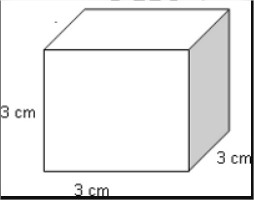# C Program To Calculate Volume Of Cube | C Programs

C Program to calculate the Volume of a Cube – In this particular article, we will detail in on the methods to evaluate the volume of a cube in C programming.

The multitude of ways to do so are as follows:

• Using Standard Method
• Using Function
• Using Pointers
• Using Macros

Several examples and sample programs have been added to this blog post as well for you. The compiler has been added for the execution of the programs.

As we all know, the cube is a universally used three-dimensional figure. A cube is a square extrapolated from to give it a height which is similar to the sides as well.

Essentially, all sides of a cube are similar. Opposites sides are parallel and all the angles of a cube are 90 degrees as well. This is how a cube looks like:As you can see, this is a cube with a side of 3 cm. The volume of a cube can be calculated with this formula:

Volume = Side^3

Hence, the volume of this cube is as follows:

Volume = 3^3 = 27 cm^3.

Thus, the methods used to calculate the volume of a cube in C programming are as follows:

## Using Standard Method

1)The value of side of a cube will store into the variable “side”.

2)The value will be substituted into the formula area=side*side*side.

3)The calculated value will store into the variable “area”, and displayed using printfunction.

output:

### Using Functions

1)The formula for the area of a cube is a=s*s*s.

2)We are calling the function float area(float s) ,using area(s).area(s) is calling function and

area(float s) is called function.

3)Then function float area(float s) return the calculated value, and that value will store into variable “s”.

output:

#### Using Pointers

1)Here *s,*v are pointer variables.

2)We are calling the function using area(&s,&v) by passing addresses of s,v.

3)The function void area(float *s,float *v) calculate the area ,that area value will store into the variable “v”.That v value will be displayed using printf statement.

output:

##### Using Macros

1)#define area(s) represents area(s) is symbolic name to the expression (s*s*s)

2)area(s)replaced with that expression given at #define.

output:
x

## C Program To Check If Alphabet, Digit or Special Character | C Programs

C program to check for an alphabet, digit or special character – In this article, ...